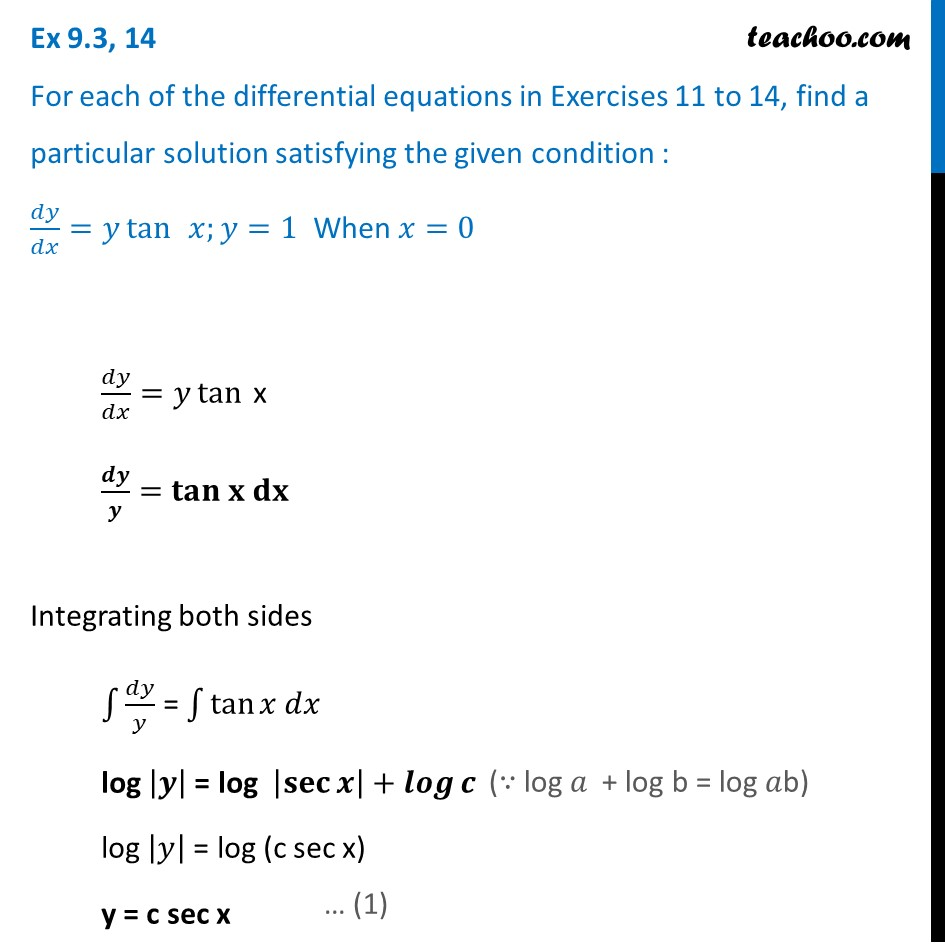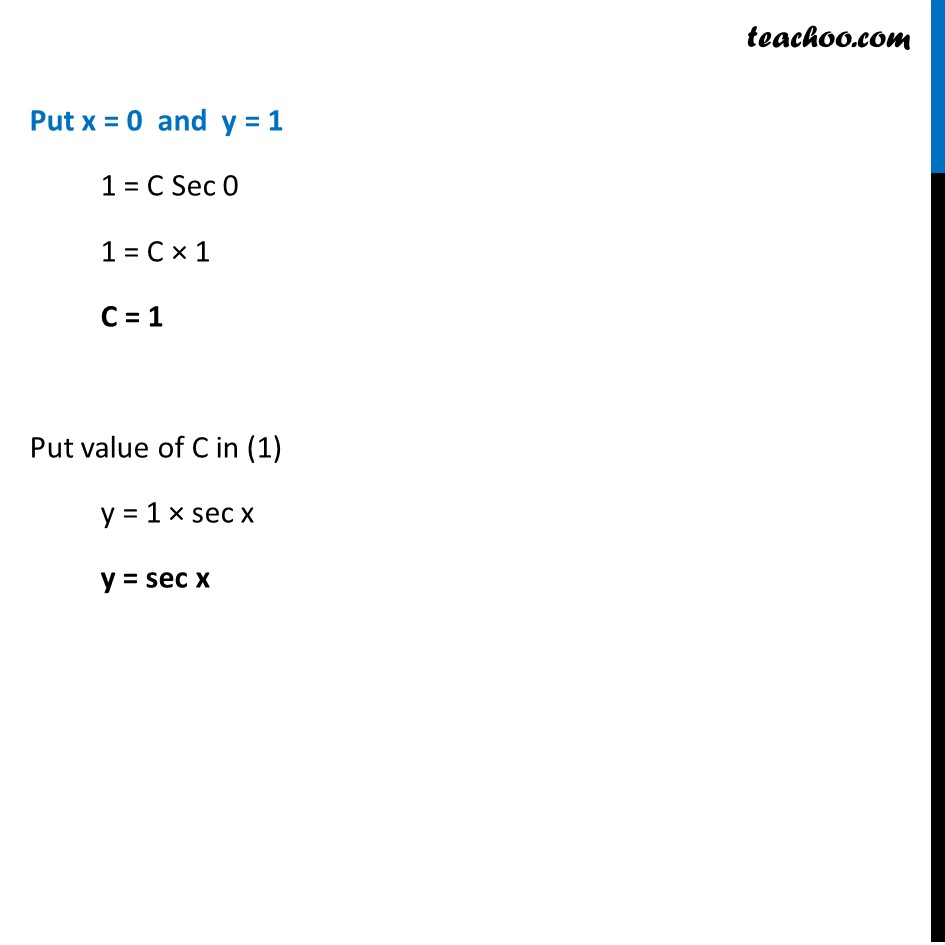Ex 9.3

Chapter 9 Class 12 Differential Equations
Serial order wiseLearn in your speed, with individual attention - Teachoo Maths 1-on-1 Class

### Transcript

Ex 9.3, 14 For each of the differential equations in Exercises 11 to 14, find a particular solution satisfying the given condition : 𝑑𝑦/𝑑𝑥=𝑦 tan⁡ 𝑥;𝑦=1 When 𝑥=0 𝑑𝑦/𝑑𝑥=𝑦 tan⁡ x 𝒅𝒚/𝒚=〖𝐭𝐚𝐧 𝐱 𝐝𝐱〗⁡ Integrating both sides ∫1▒𝑑𝑦/𝑦 = ∫1▒tan⁡〖𝑥 𝑑𝑥〗 log |𝒚| = log |𝐬𝐞𝐜⁡𝒙 |+𝒍𝒐𝒈⁡𝒄 log |𝑦| = log (c sec x) y = c sec x (∵ log 𝑎 + log b = log 𝑎b) Put x = 0 and y = 1 1 = C Sec 0 1 = C × 1 C = 1 Put value of C in (1) y = 1 × sec x y = sec x